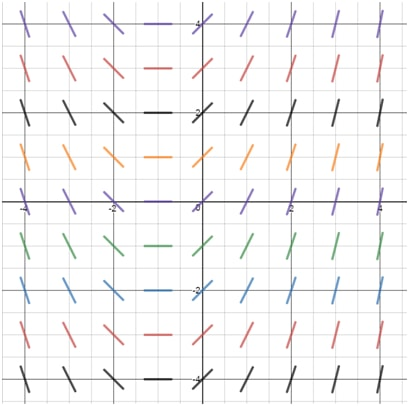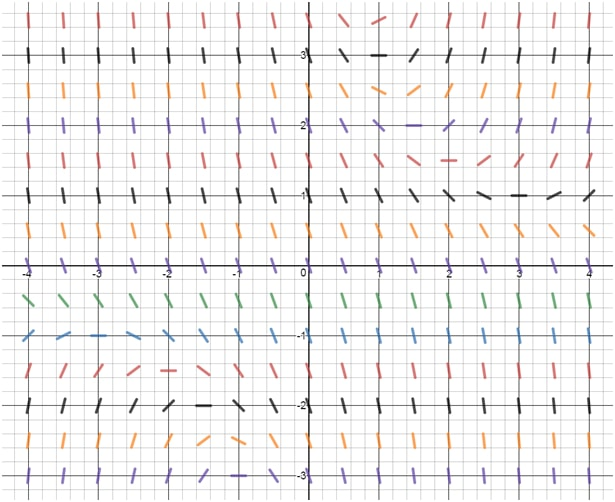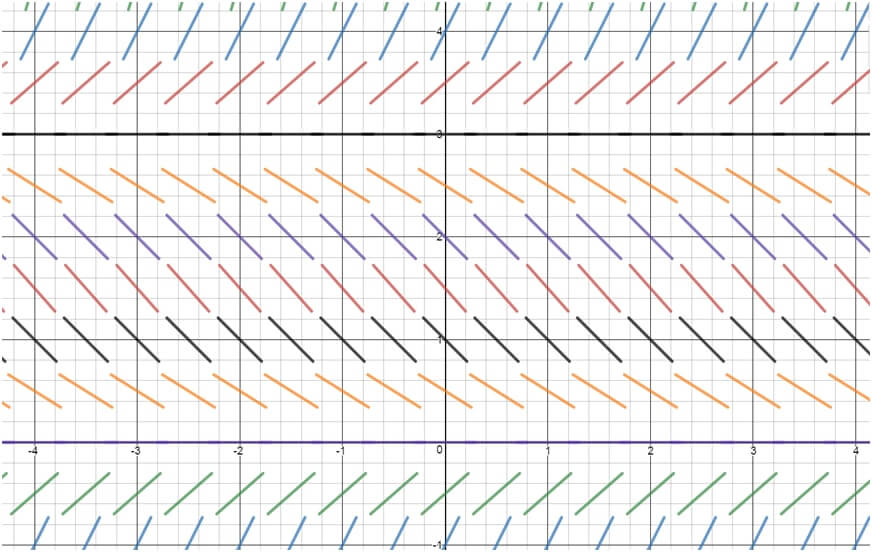# Slope fields

### Slope fields

#### Lessons

Slope fields, also called directional fields or vector fields, are graphical representations of first-order differential equations.

Slope Fields consist of a bunch of lines indicating the slope of y with respect to x, or $\frac{dy}{dx}$
• Introduction
What are Slope Fields?

• 1.
Understanding Slope Fields
Find the directional field for the following equations:
a)
$\frac{dy}{dx}=xy-x$

b)
$\frac{dy}{dx}=\frac{x^2}{(y+1)}$

• 2.
Determining the Equation from a Slope Field
Which equation best corresponds to the following slope field?i.
$\frac{dy}{dx}=y-2$
ii.
$\frac{dy}{dx}=xy-2$
iii.
$\frac{dy}{dx}=x+1$
iv.
$\frac{dy}{dx}=-x+1$

• 3.
Which equation best corresponds to the following slope field?i.
$\frac{dy}{dx}=xy-2$
ii.
$\frac{dy}{dx}=-\frac{y}{x}$
iii.
$\frac{dy}{dx}=2x+y$
iv.
$\frac{dy}{dx}=xy-3$

• 4.
Given the differential equation and its resulting slope field:
$\frac{dy}{dx}=\frac{y}{2}(y-3)$Draw a solution to the following differential equation using the following initial value conditions:
a)
$y(-2)=1$

b)
$y(0)=4$

c)
$y(1)=3$